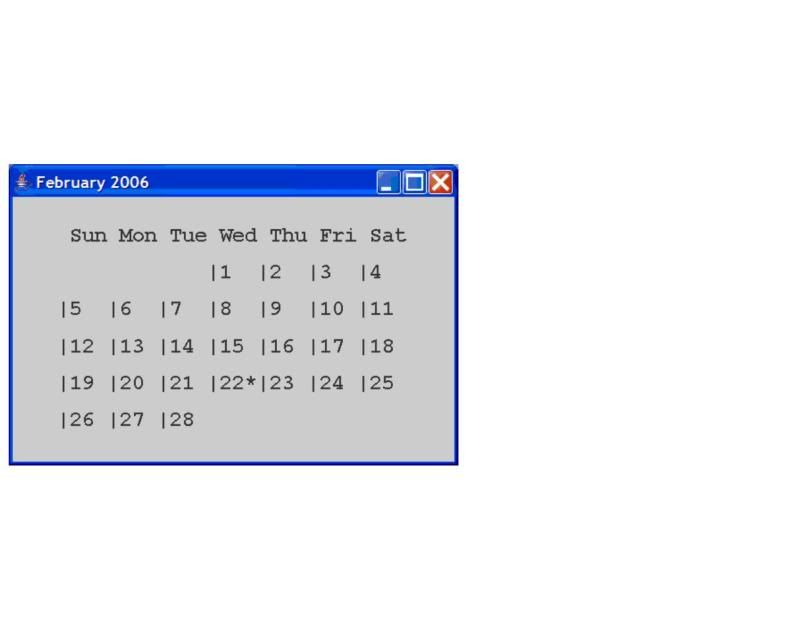# Thread: What's wrong with my code?

1.MemberJoin Date
Oct 2010
Posts
5
Rep Power
0

##What's wrong with my code?

Java Code:
```import java.awt.*;
import java.awt.font.*;
import java.awt.geom.*;
import java.text.DateFormatSymbols;
import java.util.Calendar;
import java.util.GregorianCalendar;

import javax.swing.*;

public class tester
{
public static void main(String[] args)
{
EventQueue.invokeLater(new Runnable()
{
public void run()
{
FontFrame frame = new FontFrame();
frame.setDefaultCloseOperation(JFrame.EXIT_ON_CLOSE);
frame.setVisible(true);
}
});
}
}

class FontFrame extends JFrame
{
public FontFrame()
{
setTitle("This week (2010)");
setSize(500,250);

CalendarComponent component = new CalendarComponent();
}
}

class CalendarComponent extends JPanel
{
public void paintComponent(Graphics g)
{
Graphics2D g2 = (Graphics2D) g;

GregorianCalendar d = new GregorianCalendar();
int today = d.get(Calendar.DAY_OF_MONTH);
int week = d.get(Calendar.WEEK_OF_MONTH);
int month = d.get(Calendar.MONTH);

String dates = "";
String message = "";

d.set(Calendar.DAY_OF_WEEK,1);

//print weekday name
String[] weekdayNames = new DateFormatSymbols().getShortWeekdays();

for (int i=0; i < 8; i++)
{
dates = dates + weekdayNames[i] + " ";
}

Font f = new Font("Courier", Font.BOLD, 23);
g2.setFont(f);

FontRenderContext context = g2.getFontRenderContext();
Rectangle2D bounds = f.getStringBounds(dates, context);

double x = (getWidth() - bounds.getWidth())/2;
double y = (getHeight() - bounds.getHeight())/2;

//add ascent to y to reach the baseline
double ascent = -bounds.getY();
double baseY = y + ascent;

g2.drawString(dates, (int) x, (int) baseY);

do
{
//print day
int day = d.get(Calendar.DAY_OF_MONTH);
int weekday = d.get(Calendar.DAY_OF_WEEK);
int firstDayOfWeek = d.getFirstDayOfWeek();

message = message + day;

//marks current date with *
if(day == today) message = message +"*";

if (day == day) message = message +"| ";

if (weekday == firstDayOfWeek) message = message + "\n";

}
while (d.get(Calendar.MONTH) == month);

//measure the size of the message
bounds = f.getStringBounds(message, context);

//set (x,y) = top-left of the text

x = (getWidth() - bounds.getWidth())/2;
y = (getHeight() - bounds.getHeight())/2;

//add ascent to y to reach the baseline

ascent = -bounds.getY();
baseY = y + ascent;

//draw the message

g2.drawString(message, (int) x, (int) baseY+35);
}
}```
Trying to make my program output something like this,however, I can't get the string to move to the next line for every week.
Also, the week ends a day late.
for example, the first week ends on the 7th, the second week on the 14th, and the third week on the 21st.Reply With Quote

2.MemberJoin Date
Oct 2010
Posts
63
Rep Power
0

##I assume you have probably solved this by now?Reply With Quote

newline